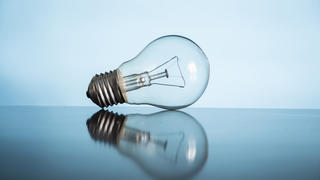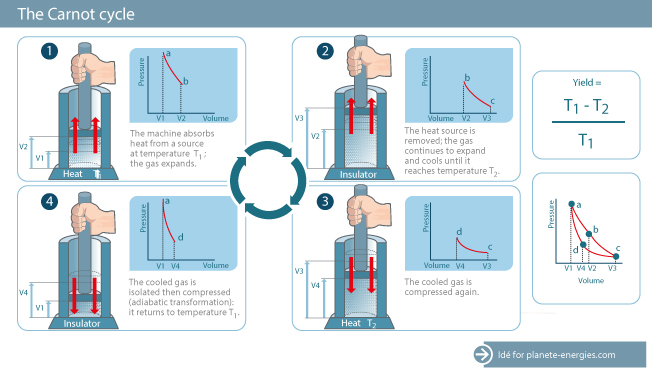# Energy: Efficiency, Power and Measurement

Published on 01.08.2015
High School

Energy transmission and transformation are governed by fundamental rules that impact our daily activities and the of the world in myriad ways.### Energy Efficiency

When one form of energy is converted into another, some of the energy initially available is dissipated, often in the form of , for example during transmission or turbine operation. Energy efficiency, or the amount of energy produced in proportion to the amount of energy used to produce it, is an important conversion-related factor. Energy efficiency is calculated by dividing the energy obtained (useful energy or energy output) by the initial energy (energy input). For example, a refrigerator has an energy efficiency of 20 to 50%, an incandescent bulb about 5%, a LED lamp over 30%, and a wind turbine 59% at most. Overall generation has an energy efficiency of about 39%.

The conversion efficiency for heating, lighting and household appliances is essential for calculating the energy efficiency of houses and buildings. The higher it is, the more energy efficient the building is likely to be — although this also depends on other factors such as insulation quality.

### Carnot Efficiency

The 19th-century physicist and engineer Nicolas Sadi Carnot determined the perfect heat engine and perfect thermodynamic cycle (known as the “Carnot cycle"). The Carnot cycle is the most efficient theoretical thermodynamic cycle for obtaining work from two constant temperature sources. Sadi Carnot demonstrated that even with a perfect machine, efficiency is always less than 100%.### Measuring Energy and Power

Energy, which is the capacity of a physical system to do work, is measured in joules (J) or -hours (Wh), with 1 watt-hour equal to 3,600 joules.

“Even with a perfect machine, energy efficiency is always less than 100%.”

Power is measured in watts. The amount of work, movement or light produced over a specific period of time determines the power of an appliance or type of energy. Power is defined as the amount of energy consumed per unit time. For example, the more powerful and brighter a light bulb is, the more it consumes over a given time period.

In economics and industry, the is used to compare different energy sources.